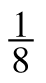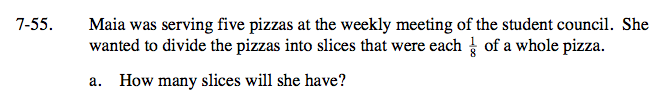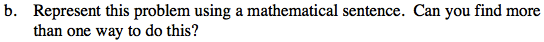### Home > CC1 > Chapter 7 > Lesson 7.2.2 > Problem7-55

7-55.
1. Maia was serving five pizzas at the weekly meeting of the student council. She wanted to divide the pizzas into slices that were eachof a whole pizza. Homework Help ✎

1. How many slices will she have?

2. Represent this problem using a mathematical sentence. Can you find more than one way to do this?$\text{How\ many\ \frac{1}{8}\ slices\ of\ pizza\ equal\ a\ whole?}$

Let x represent the number of slices in a whole pizza.

$\frac{1}{8}(x)=1$

There are 8 slices in each whole pizza.
If there are 5 whole pizzas, how many slices are there total?

40 slices$5\div\frac{1}{8}=40$